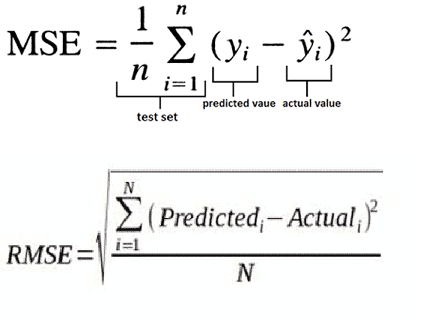All Courses

# What is RMSE and MSE in linear regression models?

##### By Ganesh, 2 years ago

How to measure accuracy using RMSE and MSE in linear regression model?

Rmse
Mse
Linear regression mpdel
##### Poornima

The Mean Squared Error (MSE) is a measure of how close a fitted line is to data points. For every data point, you take the distance vertically from the point to the corresponding y value on the curve fit (the error), and square the value. Then you add up all those values for all data points, and, in the case of a fit with two parameters such as a linear fit, divide by the number of points minus two.** The squaring is done so negative values do not cancel positive values. The smaller the Mean Squared Error, the closer the fit is to the data. The MSE has the units squared of whatever is plotted on the vertical axis.

RMSE stands for root mean square error and MSE stands for mean square error. It is just the square root of the mean square error. That is probably the most easily interpreted statistic, since it has the same units as the quantity plotted on the vertical axis.

They are the most common measures of accuracy for a linear regression model. The formulas are below.##### Elonjigar

Root Mean Squared Error using Python sklearn Library

Mean Squared Error ( MSE ) is defined as Mean or Average of the square of the difference between actual and estimated values. This means that MSE is calculated by the square of the difference between the predicted and actual target variables, divided by the number of data points. It is always non–negative values and close to zero are better.

Root Mean Squared Error is the square root of Mean Squared Error (MSE). This is the same as Mean Squared Error (MSE) but the root of the value is considered while determining the accuracy of the model.

```import numpy as np
import sklearn.metrics as metrics

actual = np.array([56,45,68,49,26,40,52,38,30,48])
predicted = np.array([58,42,65,47,29,46,50,33,31,47])
mse_sk = metrics.mean_squared_error(actual, predicted)
rmse_sk = np.sqrt(mse)
print("Root Mean Square Error :", rmse_sk)
```

#### Why You Should Learn Data Science in 2023?Jun 8th (7:00 PM) 289 Registered
More webinars

#### Related Discussions

Running random forest algorithm with one variable

View More